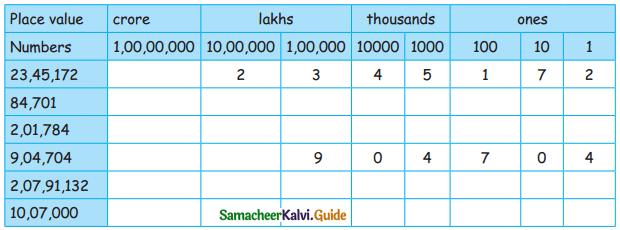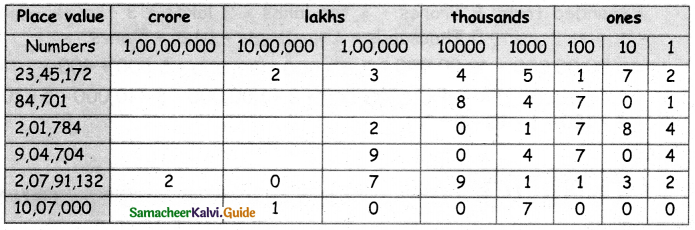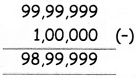Students can download 5th Maths Term 1 Chapter 2 Numbers Ex 2.2 Questions and Answers, Notes, Samacheer Kalvi 5th Maths Guide Pdf helps you to revise the complete Tamilnadu State Board New Syllabus, helps students complete homework assignments and to score high marks in board exams.

## Tamilnadu Samacheer Kalvi 5th Maths Solutions Term 1 Chapter 2 Numbers Ex 2.2

Question 1.
Consider the number 15,478

a. The place value of 7 is _______
The place value of 7 is 7 × 10 = 70

b. The place value of 4 is_______
The place value of 4 is 4 × 100 = 400

c. The place value of 1 is _______
The place value of 1 is 1 × 10,000 = 10,000Question 2.
Fill the table with the place value for the following numbers.